Скачать презентацию UNIVERSITY CERTIFICATE IN ECONOMETRICS 2017 Edition

• Количество слайдов: 55UNIVERSITY CERTIFICATE IN ECONOMETRICS 2017 · Edition 2 Course : Microeconometrics for policy evaluation Part 1 - Panel Data Models Vincent Vandenberghe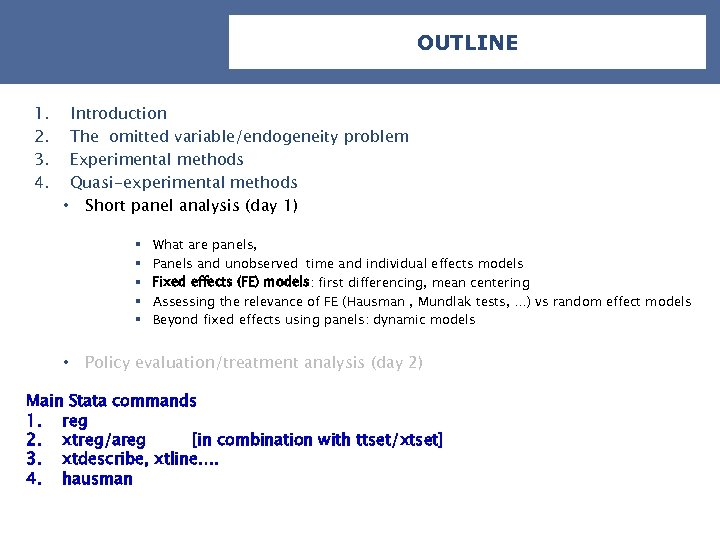OUTLINE 1. 2. 3. 4. Introduction The omitted variable/endogeneity problem Experimental methods Quasi-experimental methods • Short panel analysis (day 1) § § § • What are panels, Panels and unobserved time and individual effects models Fixed effects (FE) models: first differencing, mean centering Assessing the relevance of FE (Hausman , Mundlak tests, …) vs random effect models Beyond fixed effects using panels: dynamic models Policy evaluation/treatment analysis (day 2) Main Stata commands 1. reg 2. xtreg/areg [in combination with ttset/xtset] 3. xtdescribe, xtline…. 4. hausman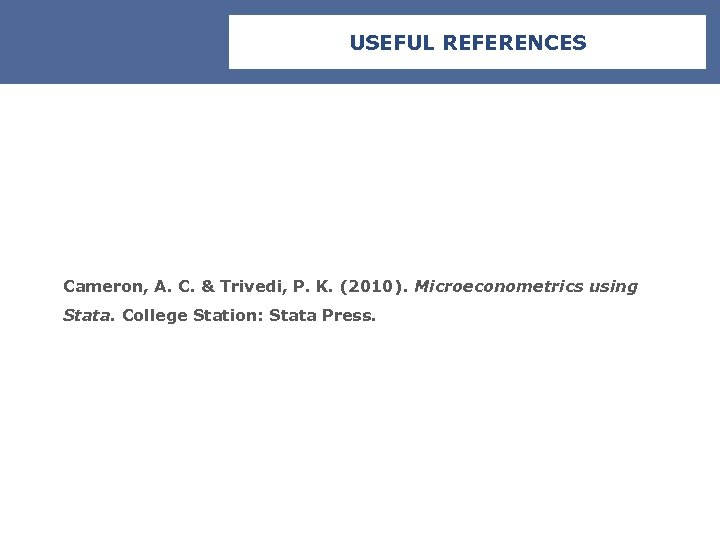USEFUL REFERENCES Cameron, A. C. & Trivedi, P. K. (2010). Microeconometrics using Stata. College Station: Stata Press.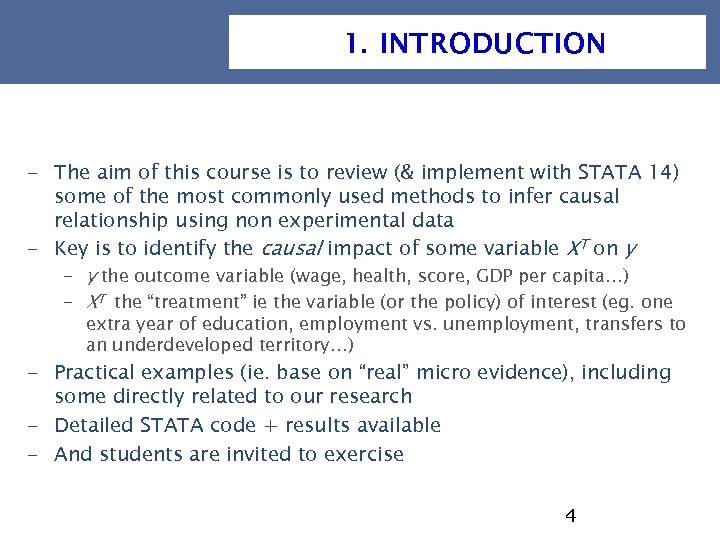1. INTRODUCTION - The aim of this course is to review (& implement with STATA 14) some of the most commonly used methods to infer causal relationship using non experimental data - Key is to identify the causal impact of some variable XT on y - y the outcome variable (wage, health, score, GDP per capita…) - XT the “treatment” ie the variable (or the policy) of interest (eg. one extra year of education, employment vs. unemployment, transfers to an underdeveloped territory…) - Practical examples (ie. base on “real” micro evidence), including some directly related to our research - Detailed STATA code + results available - And students are invited to exercise 4MATERIAL @ YOUR DISPOSAL [email protected]: LECME 2 FC TOPIC 2Panels ECcourse 1. ppt Code…Stata_code #1 EC_data. do #1 EC_Ex_corr. do #1 EC_Extra. do #1 EC_FE. do __________ (+ corrected version at the end) Data. zip the various data sets @ your disposal via the web: https: //perso. uclouvain. be/vincent. vandenberghe/Stata_EC 1. html 5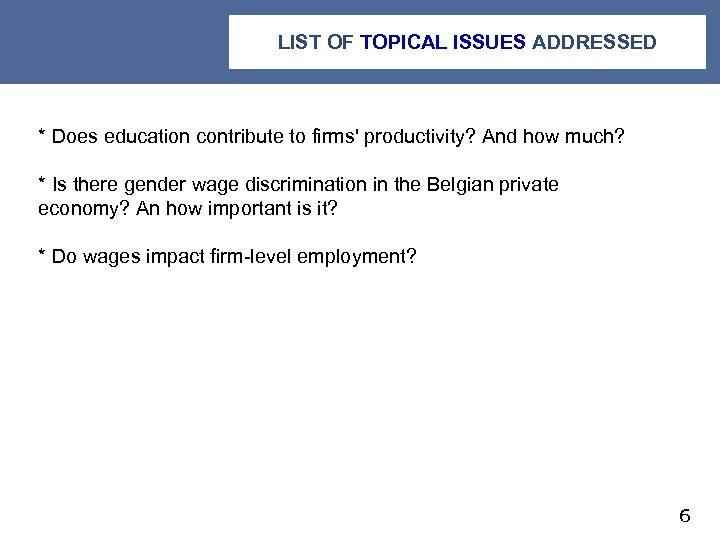LIST OF TOPICAL ISSUES ADDRESSED * Does education contribute to firms' productivity? And how much? * Is there gender wage discrimination in the Belgian private economy? An how important is it? * Do wages impact firm-level employment? 6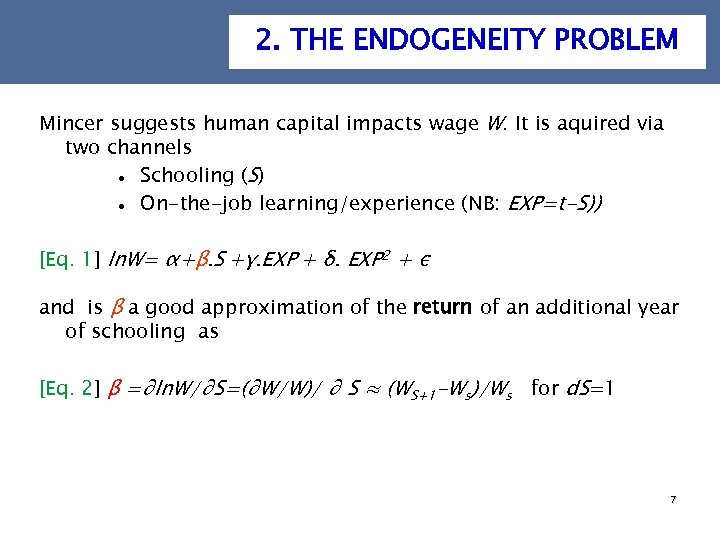2. THE ENDOGENEITY PROBLEM Mincer suggests human capital impacts wage W. It is aquired via two channels Schooling (S) On-the-job learning/experience (NB: EXP=t-S)) [Eq. 1] ln. W= α+β. S +γ. EXP + δ. EXP 2 + ε and is β a good approximation of the return of an additional year of schooling as [Eq. 2] β =∂ln. W/∂S=(∂W/W)/ ∂ S ≈ (WS+1 -Ws)/Ws for d. S=1 7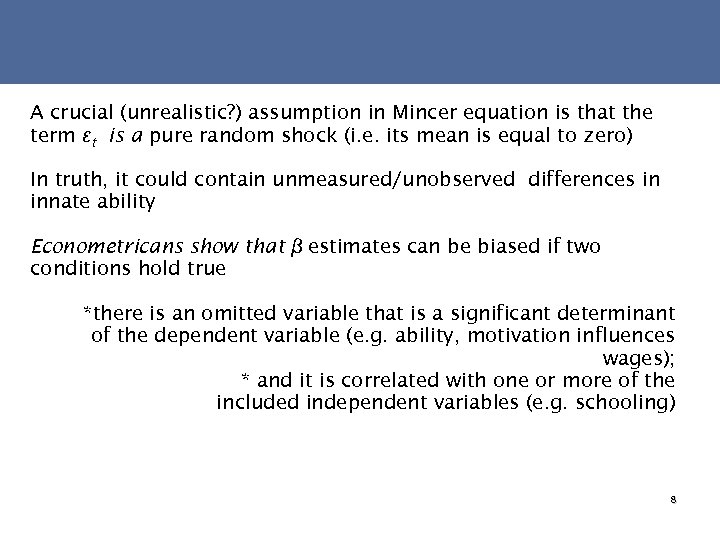A crucial (unrealistic? ) assumption in Mincer equation is that the term εt is a pure random shock (i. e. its mean is equal to zero) In truth, it could contain unmeasured/unobserved differences in innate ability Econometricans show that β estimates can be biased if two conditions hold true *there is an omitted variable that is a significant determinant of the dependent variable (e. g. ability, motivation influences wages); * and it is correlated with one or more of the included independent variables (e. g. schooling) 8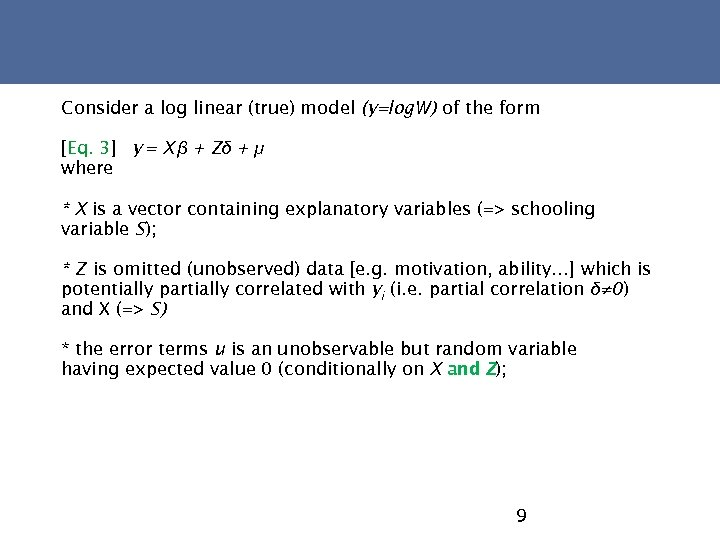Consider a log linear (true) model (y=log. W) of the form [Eq. 3] y = X β + Zδ + μ where * X is a vector containing explanatory variables (=> schooling variable S); * Z is omitted (unobserved) data [e. g. motivation, ability…] which is potentially partially correlated with yi (i. e. partial correlation δ≠ 0) and X (=> S) * the error terms u is an unobservable but random variable having expected value 0 (conditionally on X and Z); 9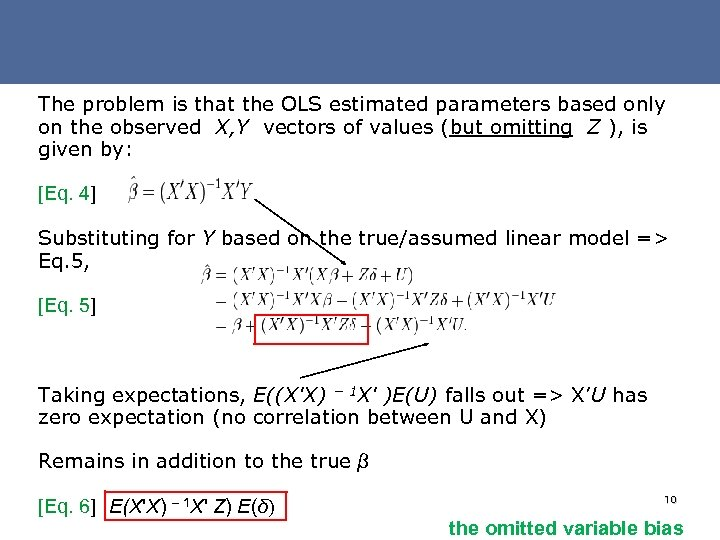The problem is that the OLS estimated parameters based only on the observed X, Y vectors of values (but omitting Z ), is given by: [Eq. 4] Substituting for Y based on the true/assumed linear model => Eq. 5, [Eq. 5] Taking expectations, E((X'X) − 1 X' )E(U) falls out => X’U has zero expectation (no correlation between U and X) Remains in addition to the true β [Eq. 6] E(X'X) – 1 X' Z) E(δ) 10 the omitted variable bias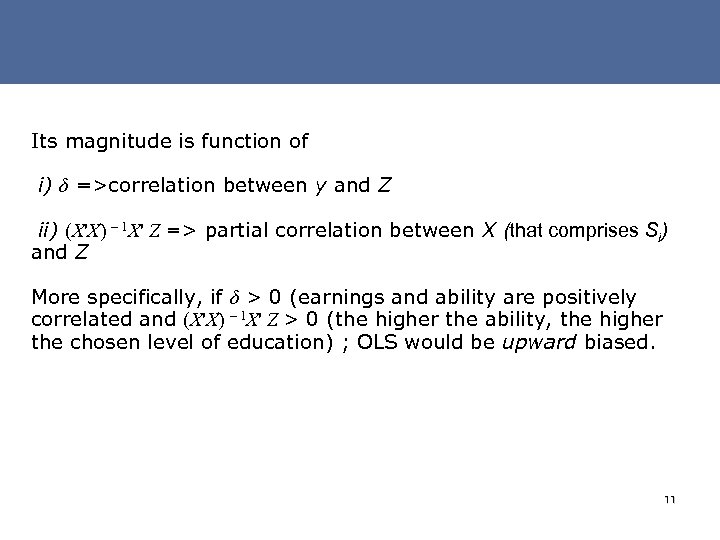Its magnitude is function of i) δ =>correlation between y and Z ii) (X'X) – 1 X' Z => partial correlation between X (that comprises Si) and Z More specifically, if δ > 0 (earnings and ability are positively correlated and (X'X) – 1 X' Z > 0 (the higher the ability, the higher the chosen level of education) ; OLS would be upward biased. 113. EXPERIMENTAL METHODS Experimental research design offer the most plausibly unbiased estimates But experiments are frequently infeasible due to cost or moral objections – e. g no one proposes to randomly assign smoking to individuals to assess health risks or to randomly assign divorce status to parents so as to measure the impacts on their children 12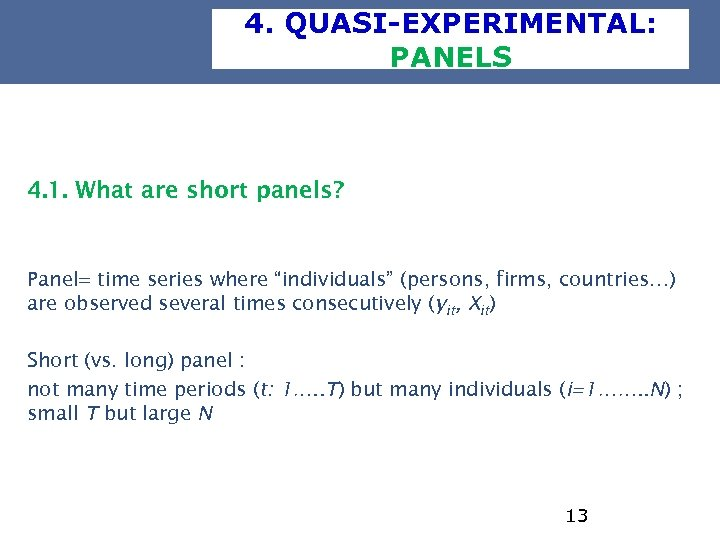4. QUASI-EXPERIMENTAL: PANELS 4. 1. What are short panels? Panel= time series where “individuals” (persons, firms, countries…) are observed several times consecutively (yit, Xit) Short (vs. long) panel : not many time periods (t: 1…. . T) but many individuals (i=1……. . N) ; small T but large N 13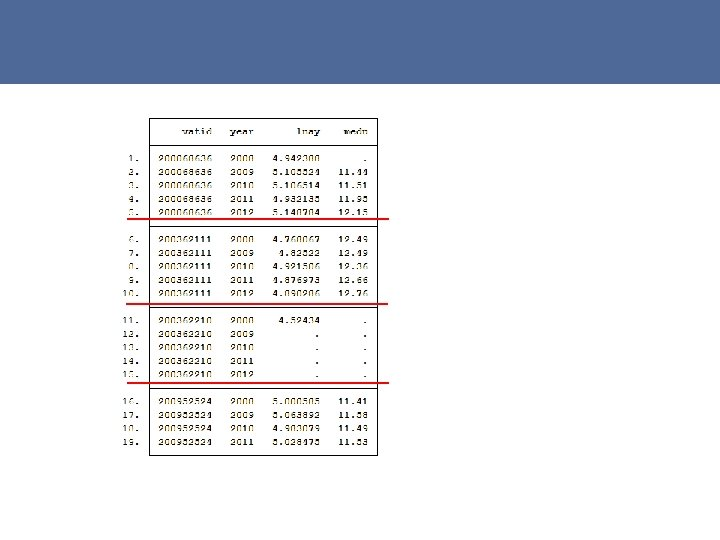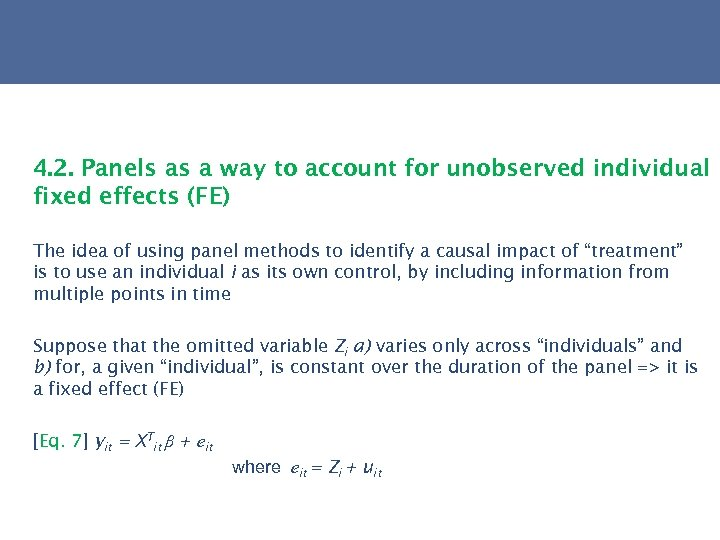4. 2. Panels as a way to account for unobserved individual fixed effects (FE) The idea of using panel methods to identify a causal impact of “treatment” is to use an individual i as its own control, by including information from multiple points in time Suppose that the omitted variable Zi a) varies only across “individuals” and b) for, a given “individual”, is constant over the duration of the panel => it is a fixed effect (FE) [Eq. 7] yit = XTit β + eit where eit = Zi + uit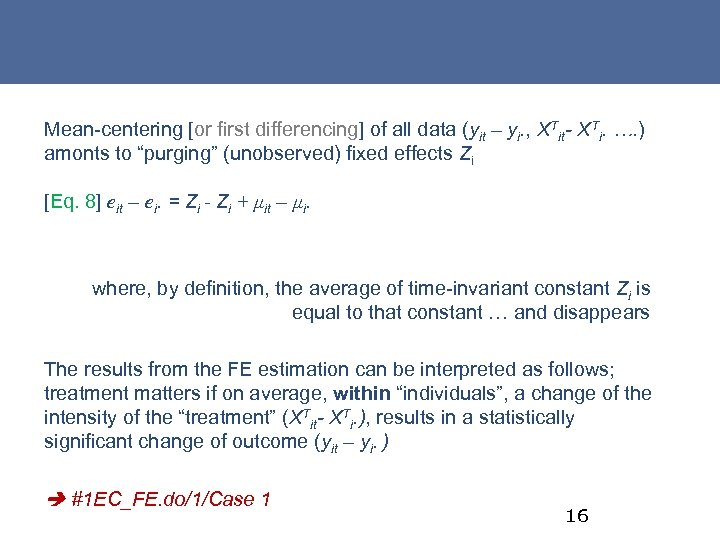Mean-centering [or first differencing] of all data (yit – yi. , XTit- XTi. …. ) amonts to “purging” (unobserved) fixed effects Zi [Eq. 8] eit – ei. = Zi - Zi + μit – μi. where, by definition, the average of time-invariant constant Zi is equal to that constant … and disappears The results from the FE estimation can be interpreted as follows; treatment matters if on average, within “individuals”, a change of the intensity of the “treatment” (XTit- XTi. ), results in a statistically significant change of outcome (yit – yi. ) #1 EC_FE. do/1/Case 1 16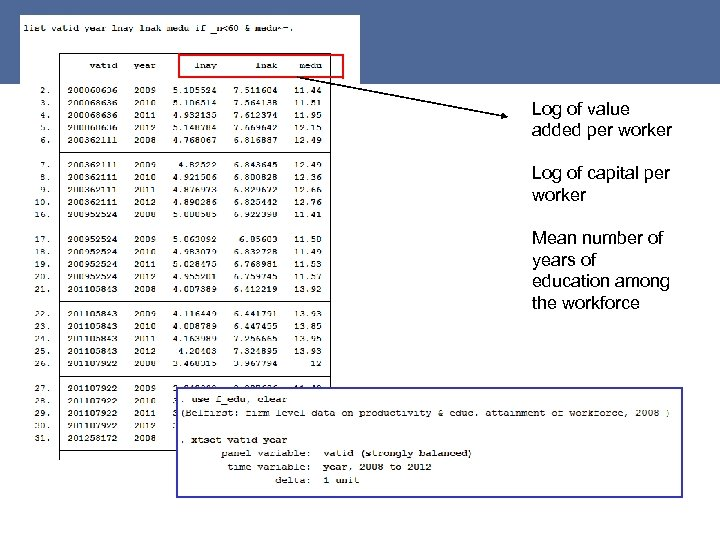Log of value added per worker Log of capital per worker Mean number of years of education among the workforce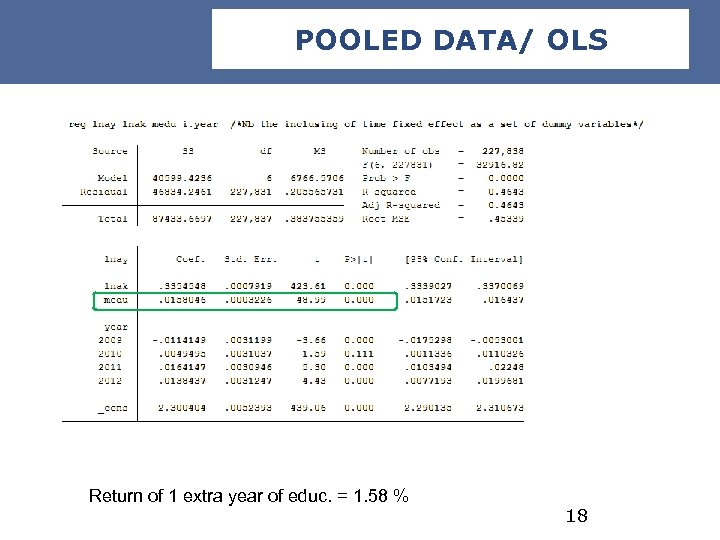POOLED DATA/ OLS Return of 1 extra year of educ. = 1. 58 % 18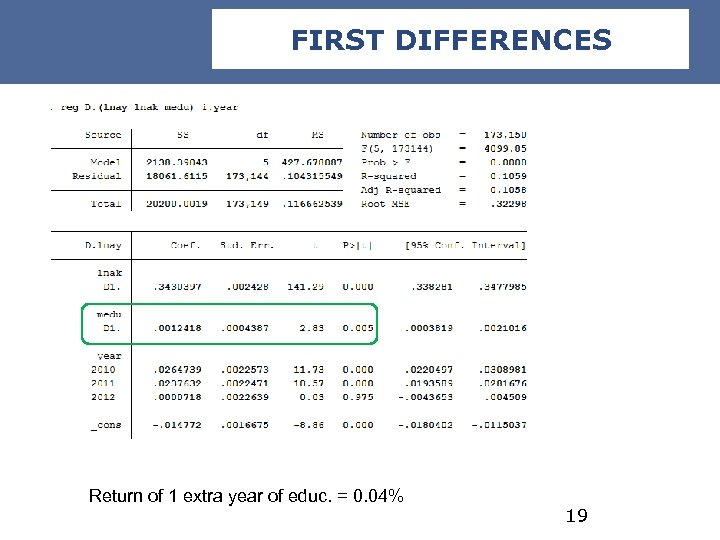FIRST DIFFERENCES Return of 1 extra year of educ. = 0. 04% 19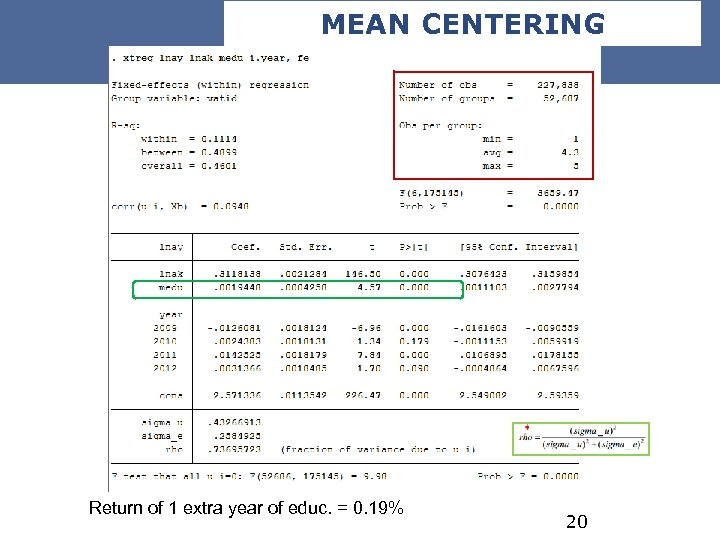MEAN CENTERING Return of 1 extra year of educ. = 0. 19% 20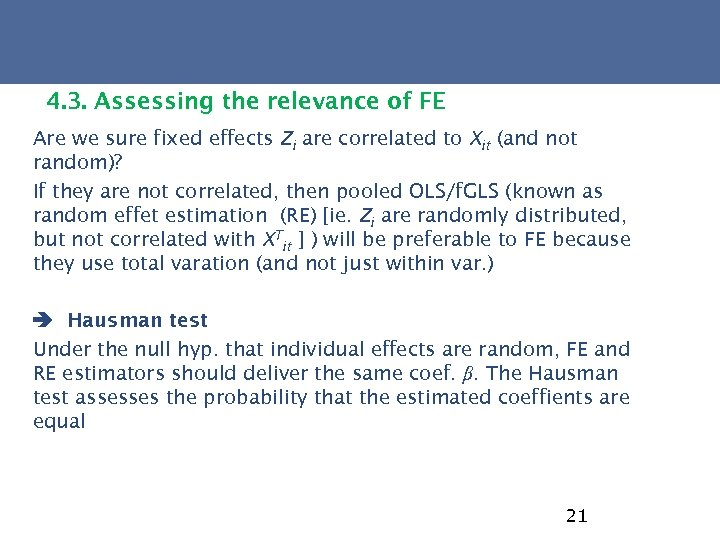4. 3. Assessing the relevance of FE Are we sure fixed effects Zi are correlated to Xit (and not random)? If they are not correlated, then pooled OLS/f. GLS (known as random effet estimation (RE) [ie. Zi are randomly distributed, but not correlated with XTit ] ) will be preferable to FE because they use total varation (and not just within var. ) Hausman test Under the null hyp. that individual effects are random, FE and RE estimators should deliver the same coef. β. The Hausman test assesses the probability that the estimated coeffients are equal 21https: //www. youtube. com/watch? v=54 o 4 -b. N 9 By 4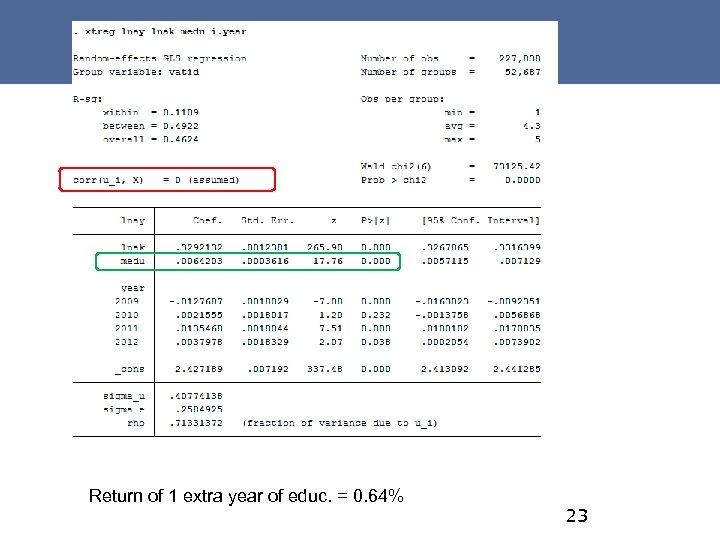Return of 1 extra year of educ. = 0. 64% 23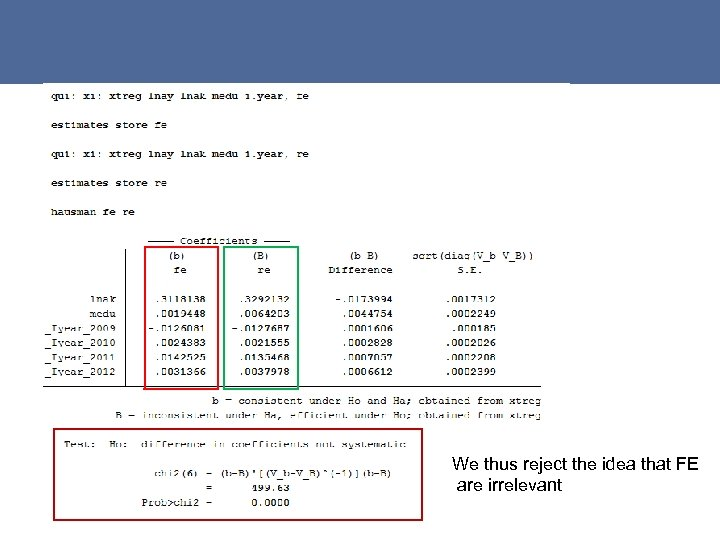We thus reject the idea that FE are irrelevant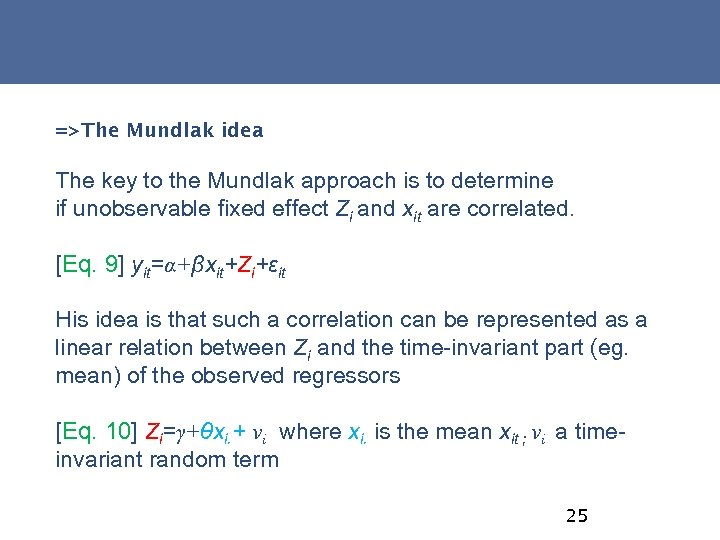=>The Mundlak idea The key to the Mundlak approach is to determine if unobservable fixed effect Zi and xit are correlated. [Eq. 9] yit=α+βxit+Zi+εit His idea is that such a correlation can be represented as a linear relation between Zi and the time-invariant part (eg. mean) of the observed regressors [Eq. 10] Zi=γ+θxi. + νi where xi. is the mean xit ; νi a timeinvariant random term 25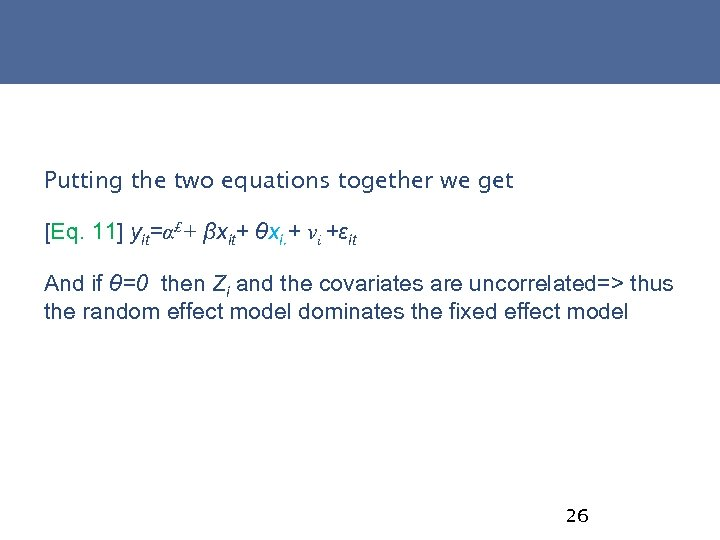Putting the two equations together we get [Eq. 11] yit=α£+ βxit+ θxi. + νi +εit And if θ=0 then Zi and the covariates are uncorrelated=> thus the random effect model dominates the fixed effect model 26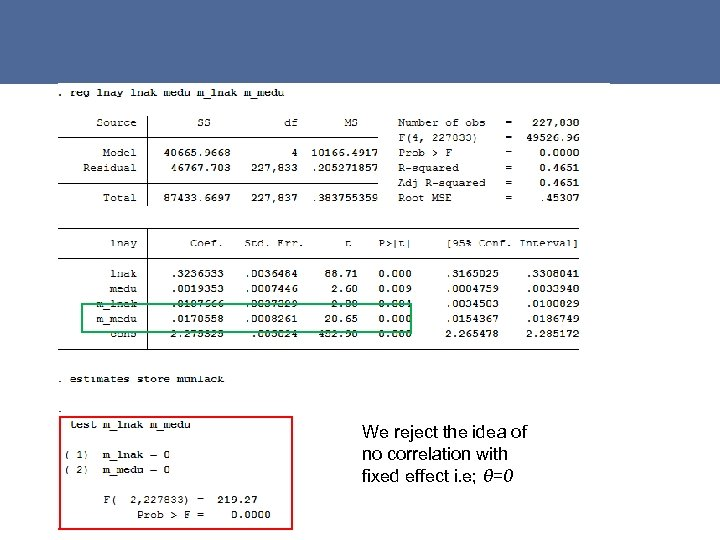We reject the idea of no correlation with fixed effect i. e; θ=0 27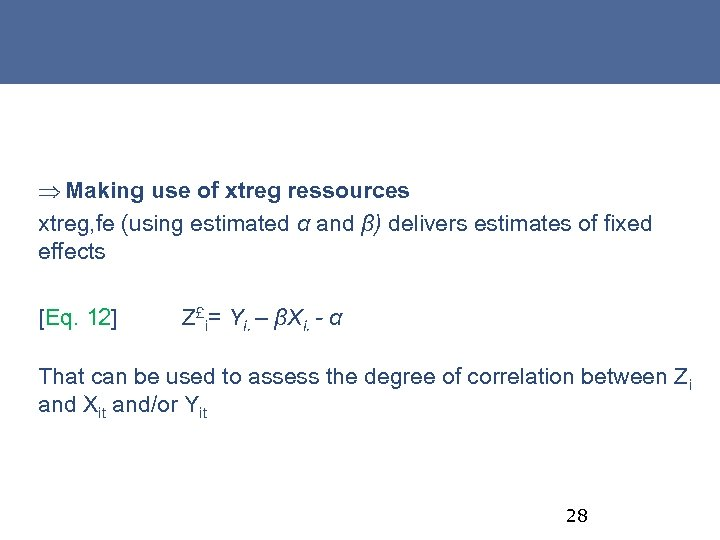Þ Making use of xtreg ressources xtreg, fe (using estimated α and β) delivers estimates of fixed effects [Eq. 12] Z£i= Yi. – βXi. - α That can be used to assess the degree of correlation between Zi and Xit and/or Yit 28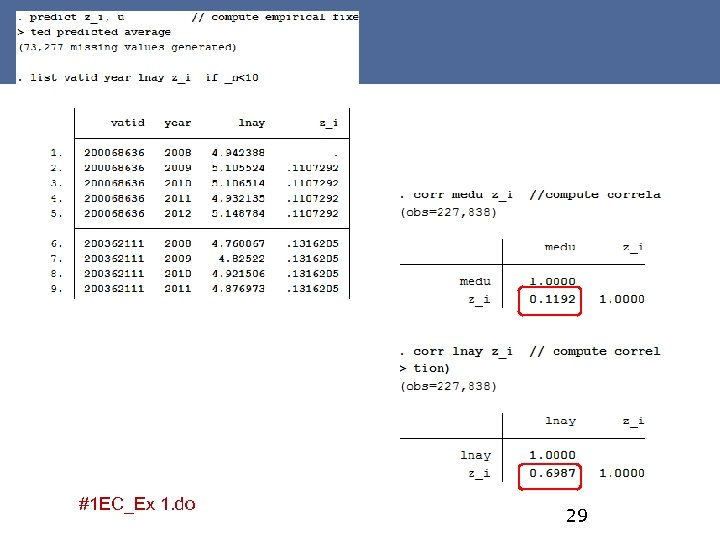#1 EC_Ex 1. do 29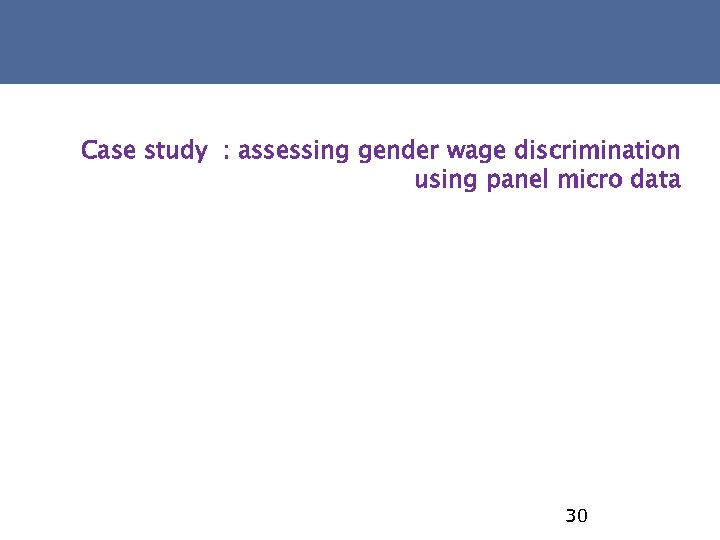Case study : assessing gender wage discrimination using panel micro data 30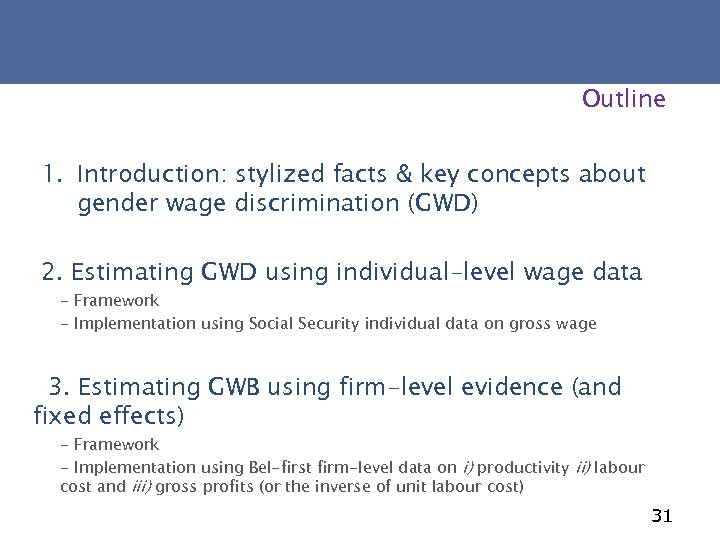Outline 1. Introduction: stylized facts & key concepts about gender wage discrimination (GWD) 2. Estimating GWD using individual-level wage data - Framework - Implementation using Social Security individual data on gross wage 3. Estimating GWB using firm-level evidence (and fixed effects) - Framework - Implementation using Bel-first firm-level data on i) productivity ii) labour cost and iii) gross profits (or the inverse of unit labour cost) 31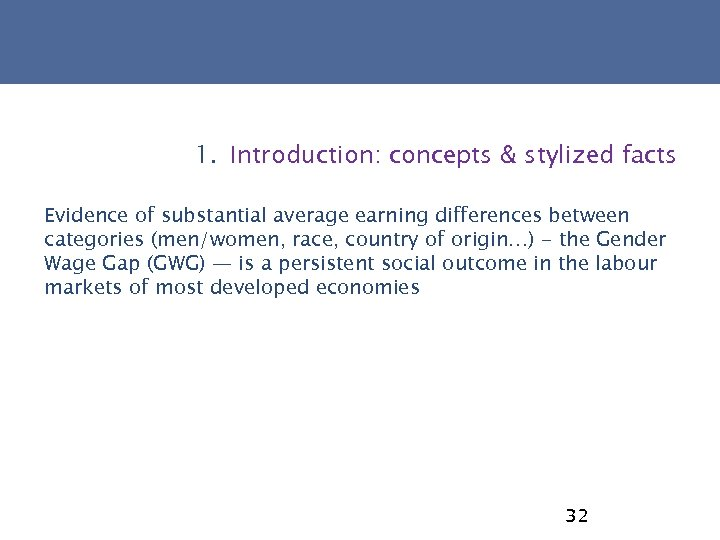1. Introduction: concepts & stylized facts Evidence of substantial average earning differences between categories (men/women, race, country of origin…) - the Gender Wage Gap (GWG) — is a persistent social outcome in the labour markets of most developed economies 32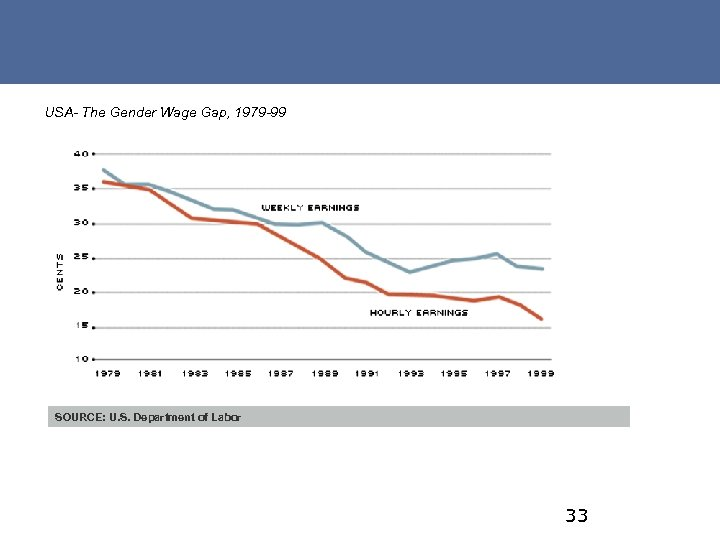USA- The Gender Wage Gap, 1979 -99 SOURCE: U. S. Department of Labor 33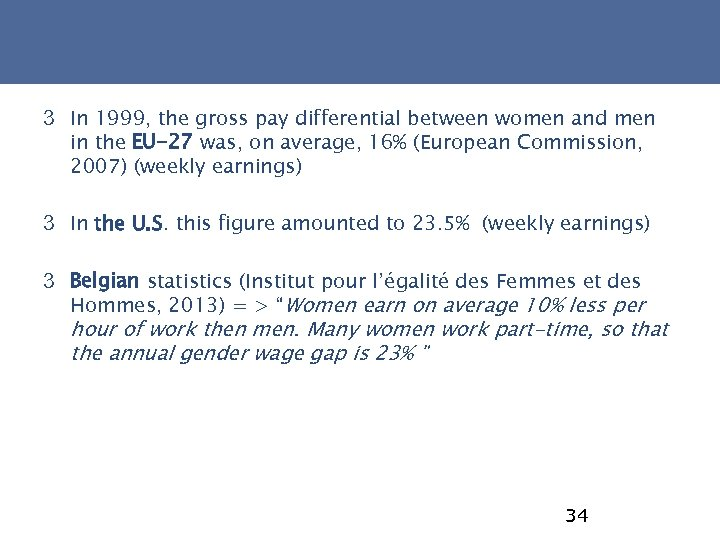3 In 1999, the gross pay differential between women and men in the EU-27 was, on average, 16% (European Commission, 2007) (weekly earnings) 3 In the U. S. this figure amounted to 23. 5% (weekly earnings) 3 Belgian statistics (Institut pour l’égalité des Femmes et des Hommes, 2013) = > “Women earn on average 10% less per hour of work then men. Many women work part-time, so that the annual gender wage gap is 23% " 34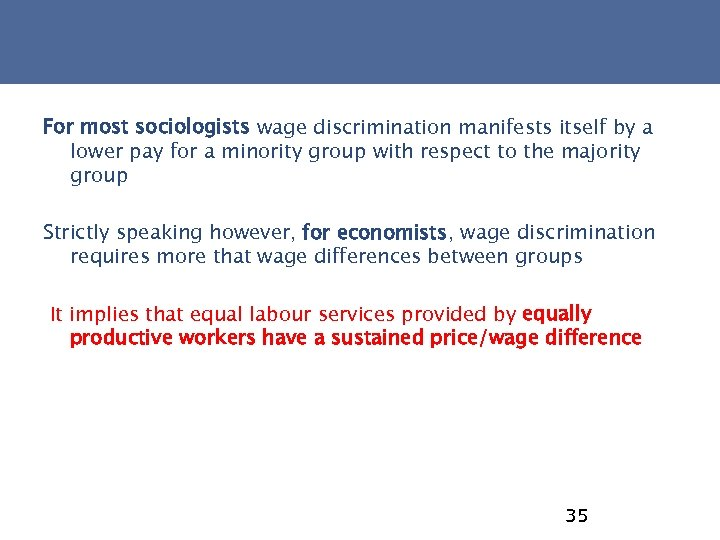For most sociologists wage discrimination manifests itself by a lower pay for a minority group with respect to the majority group Strictly speaking however, for economists, wage discrimination requires more that wage differences between groups It implies that equal labour services provided by equally productive workers have a sustained price/wage difference 35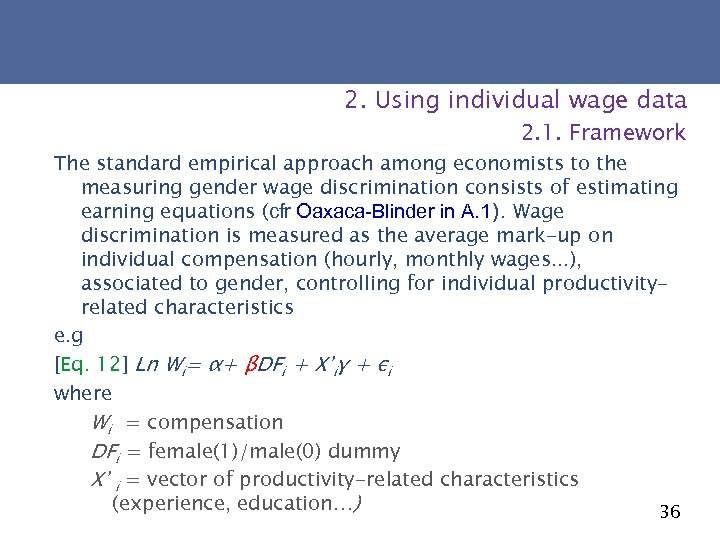2. Using individual wage data 2. 1. Framework The standard empirical approach among economists to the measuring gender wage discrimination consists of estimating earning equations (cfr Oaxaca-Blinder in A. 1). Wage discrimination is measured as the average mark-up on individual compensation (hourly, monthly wages. . . ), associated to gender, controlling for individual productivityrelated characteristics e. g [Eq. 12] Ln Wi= α+ βDFi + X’iγ + εi where Wi = compensation DFi = female(1)/male(0) dummy X’ i = vector of productivity-related characteristics (experience, education…) 36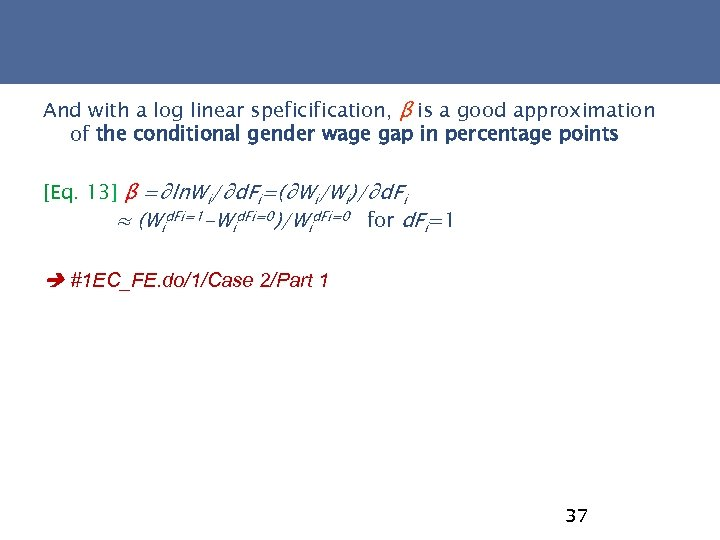And with a log linear speficification, β is a good approximation of the conditional gender wage gap in percentage points [Eq. 13] β =∂ln. Wi/∂d. Fi=(∂Wi/Wi)/∂d. Fi ≈ (Wid. Fi=1 -Wid. Fi=0)/Wid. Fi=0 for d. Fi=1 #1 EC_FE. do/1/Case 2/Part 1 37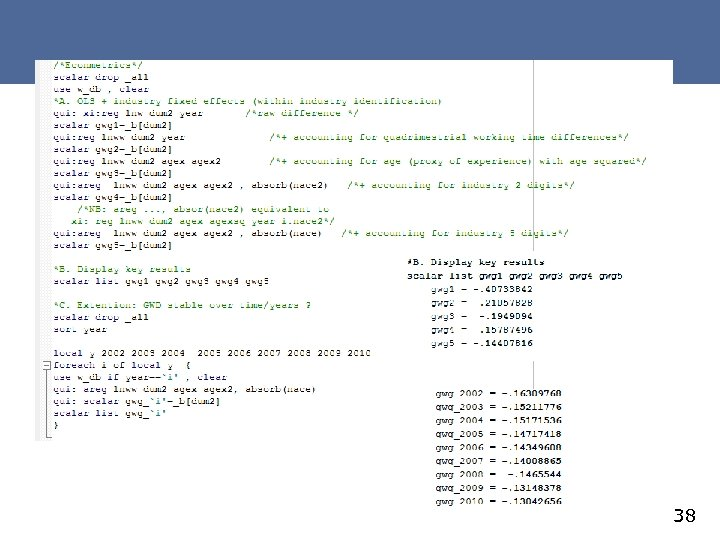38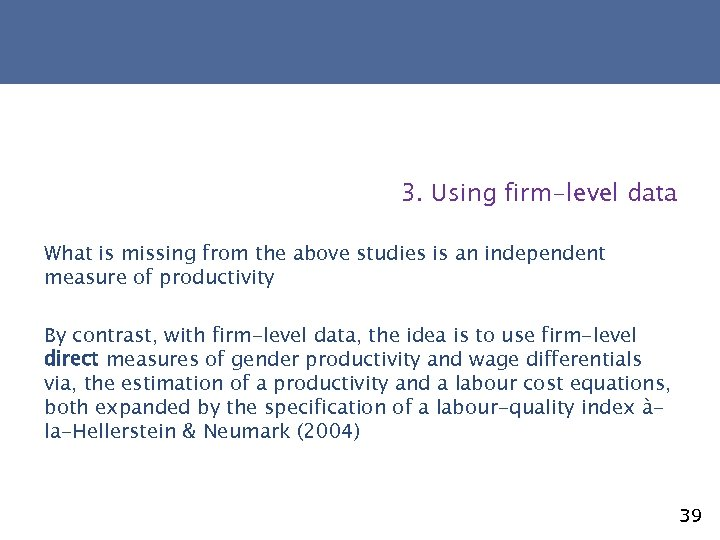3. Using firm-level data What is missing from the above studies is an independent measure of productivity By contrast, with firm-level data, the idea is to use firm-level direct measures of gender productivity and wage differentials via, the estimation of a productivity and a labour cost equations, both expanded by the specification of a labour-quality index àla-Hellerstein & Neumark (2004) 39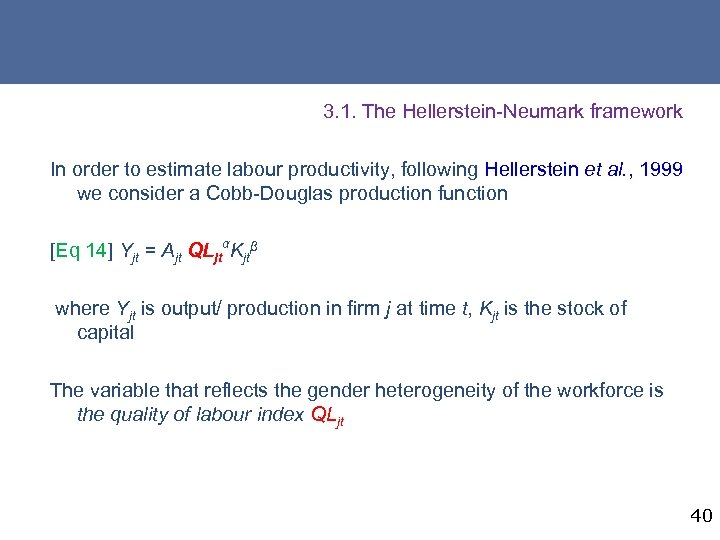3. 1. The Hellerstein-Neumark framework In order to estimate labour productivity, following Hellerstein et al. , 1999 we consider a Cobb-Douglas production function [Eq 14] Yjt = Ajt QLjtαKjtβ where Yjt is output/ production in firm j at time t, Kjt is the stock of capital The variable that reflects the gender heterogeneity of the workforce is the quality of labour index QLjt 40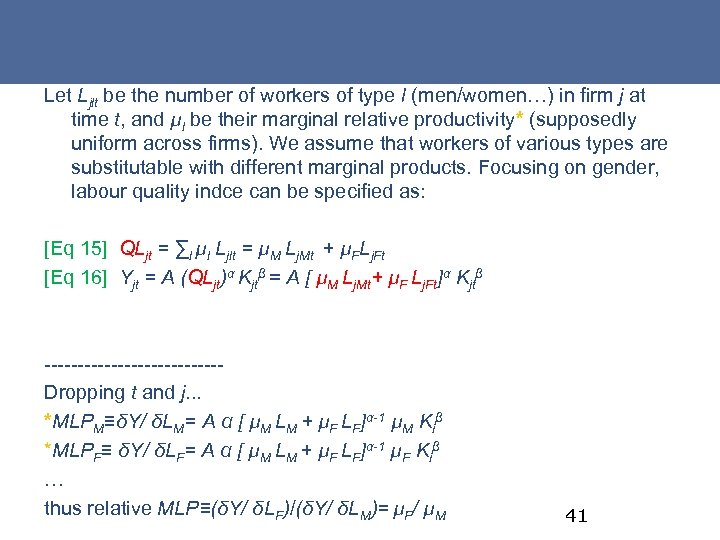Let Ljlt be the number of workers of type l (men/women…) in firm j at time t, and µl be their marginal relative productivity* (supposedly uniform across firms). We assume that workers of various types are substitutable with different marginal products. Focusing on gender, labour quality indce can be specified as: [Eq 15] QLjt = ∑l µl Ljlt = µM Lj. Mt + µFLj. Ft [Eq 16] Yjt = A (QLjt)α Kjtβ = A [ µM Lj. Mt+ µF Lj. Ft]α Kjtβ -------------Dropping t and j. . . *MLPM≡δY/ δLM= A α [ µM LM + µF LF]α-1 µM Kiβ *MLPF≡ δY/ δLF= A α [ µM LM + µF LF]α-1 µF Kiβ … thus relative MLP≡(δY/ δLF)/(δY/ δLM)= µF/ µM 41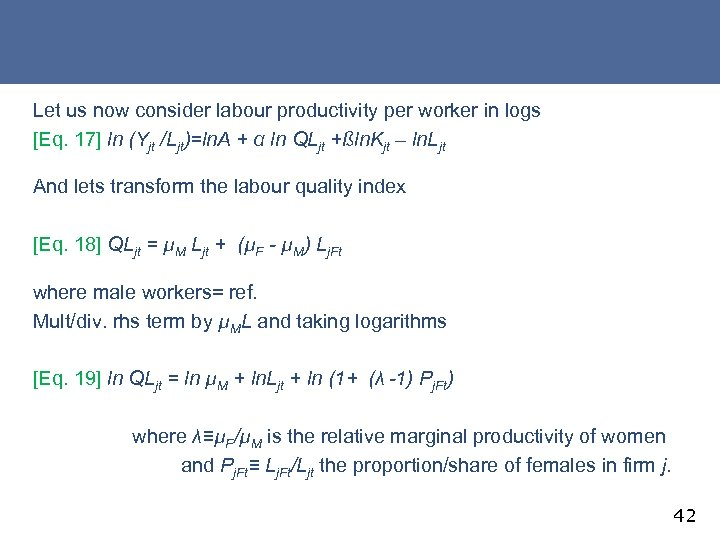Let us now consider labour productivity per worker in logs [Eq. 17] ln (Yjt /Ljt)=ln. A + α ln QLjt +ßln. Kjt – ln. Ljt And lets transform the labour quality index [Eq. 18] QLjt = µM Ljt + (µF - µM) Lj. Ft where male workers= ref. Mult/div. rhs term by µML and taking logarithms [Eq. 19] ln QLjt = ln µM + ln. Ljt + ln (1+ (λ -1) Pj. Ft) where λ≡µF/µM is the relative marginal productivity of women and Pj. Ft≡ Lj. Ft/Ljt the proportion/share of females in firm j. 42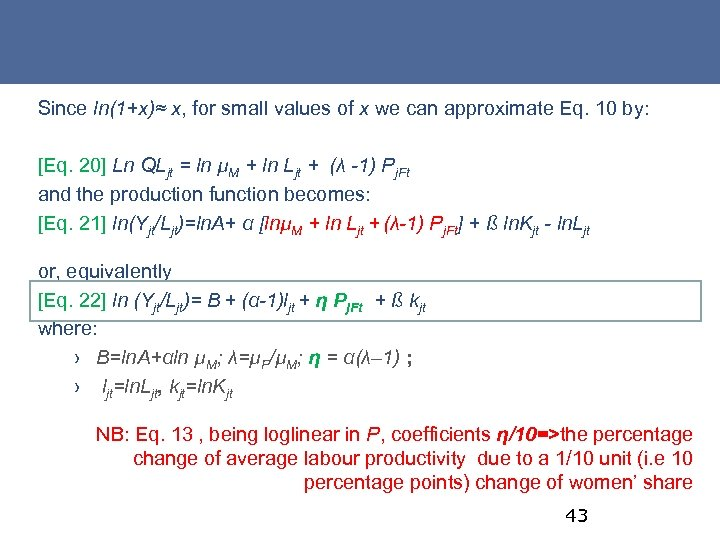Since ln(1+x)≈ x, for small values of x we can approximate Eq. 10 by: [Eq. 20] Ln QLjt = ln µM + ln Ljt + (λ -1) Pj. Ft and the production function becomes: [Eq. 21] ln(Yjt/Ljt)=ln. A+ α [lnµM + ln Ljt + (λ-1) Pj. Ft] + ß ln. Kjt - ln. Ljt or, equivalently [Eq. 22] ln (Yjt/Ljt)= B + (α-1)ljt + η Pj. Ft + ß kjt where: › B=ln. A+αln µM; λ=µF/µM; η = α(λ– 1) ; › ljt=ln. Ljt, kjt=ln. Kjt NB: Eq. 13 , being loglinear in P, coefficients η/10=>the percentage change of average labour productivity due to a 1/10 unit (i. e 10 percentage points) change of women’ share 43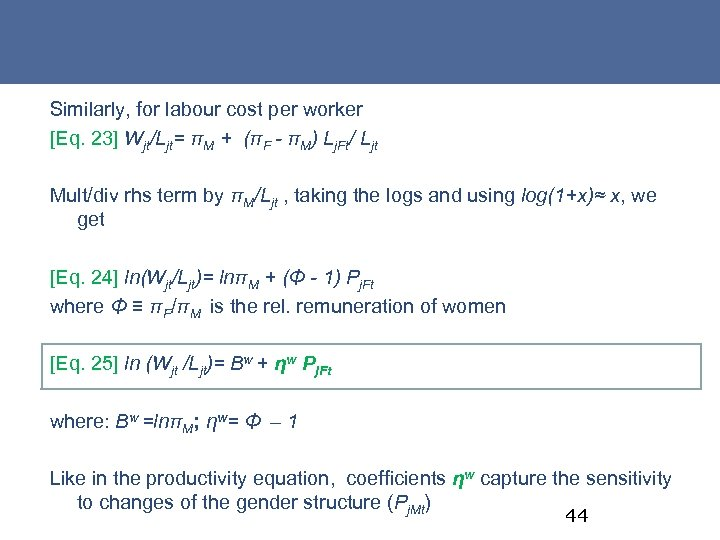Similarly, for labour cost per worker [Eq. 23] Wjt/Ljt= πM + (πF - πM) Lj. Ft/ Ljt Mult/div rhs term by πM/Ljt , taking the logs and using log(1+x)≈ x, we get [Eq. 24] ln(Wjt/Ljt)= lnπM + (Φ - 1) Pj. Ft where Φ ≡ πF/πM is the rel. remuneration of women [Eq. 25] ln (Wjt /Ljt)= Bw + ηw Pj. Ft where: Bw =lnπM; ηw= Φ – 1 Like in the productivity equation, coefficients ηw capture the sensitivity to changes of the gender structure (Pj. Mt) 44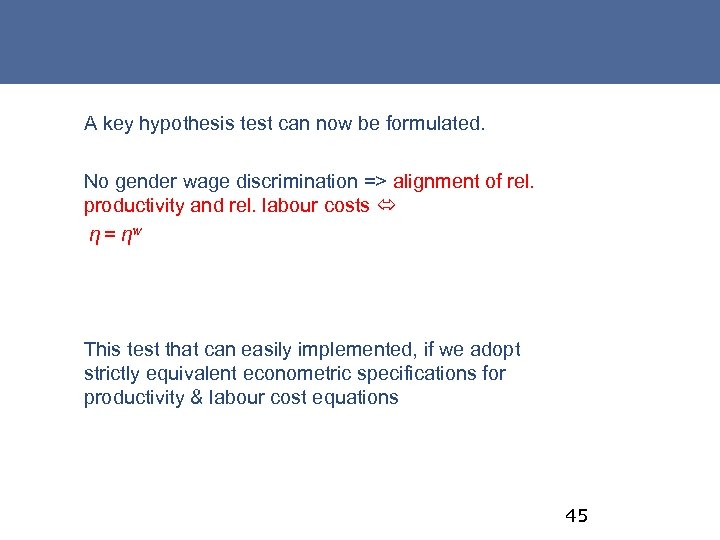A key hypothesis test can now be formulated. No gender wage discrimination => alignment of rel. productivity and rel. labour costs η = ηw This test that can easily implemented, if we adopt strictly equivalent econometric specifications for productivity & labour cost equations 45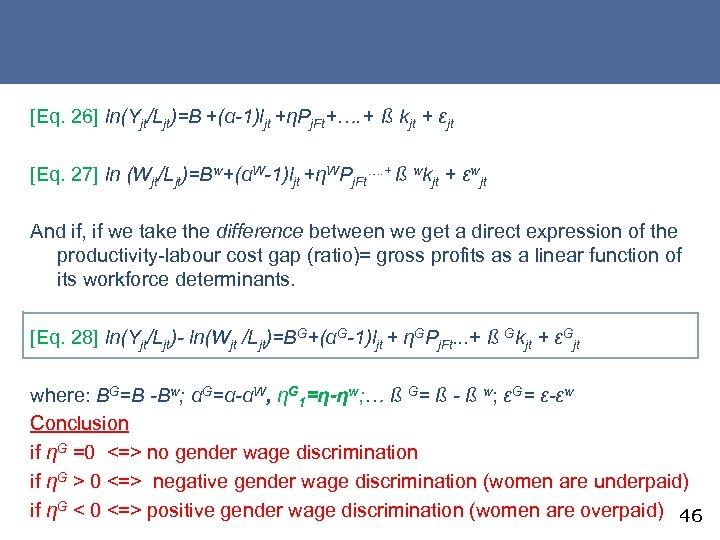[Eq. 26] ln(Yjt/Ljt)=B +(α-1)ljt +ηPj. Ft+…. + ß kjt + εjt [Eq. 27] ln (Wjt/Ljt)=Bw+(αW-1)ljt +ηWPj. Ft…. + ß wkjt + εwjt And if, if we take the difference between we get a direct expression of the productivity-labour cost gap (ratio)= gross profits as a linear function of its workforce determinants. [Eq. 28] ln(Yjt/Ljt)- ln(Wjt /Ljt)=BG+(αG-1)ljt + ηGPj. Ft. . . + ß Gkjt + εGjt where: BG=B -Bw; αG=α-αW, ηG 1=η-ηw; … ß G= ß - ß w; εG= ε-εw Conclusion if ηG =0 <=> no gender wage discrimination if ηG > 0 <=> negative gender wage discrimination (women are underpaid) if ηG < 0 <=> positive gender wage discrimination (women are overpaid) 46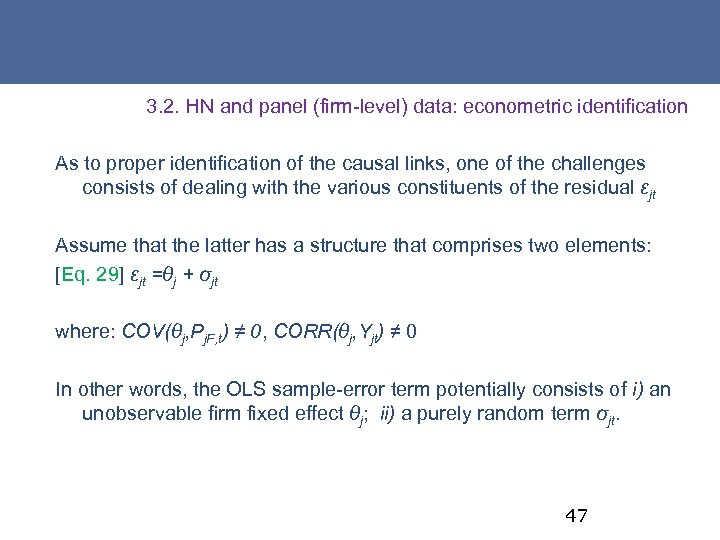3. 2. HN and panel (firm-level) data: econometric identification As to proper identification of the causal links, one of the challenges consists of dealing with the various constituents of the residual εjt Assume that the latter has a structure that comprises two elements: [Eq. 29] εjt =θj + σjt where: COV(θj, Pj. F, t) ≠ 0, CORR(θj, Yjt) ≠ 0 In other words, the OLS sample-error term potentially consists of i) an unobservable firm fixed effect θj; ii) a purely random term σjt. 47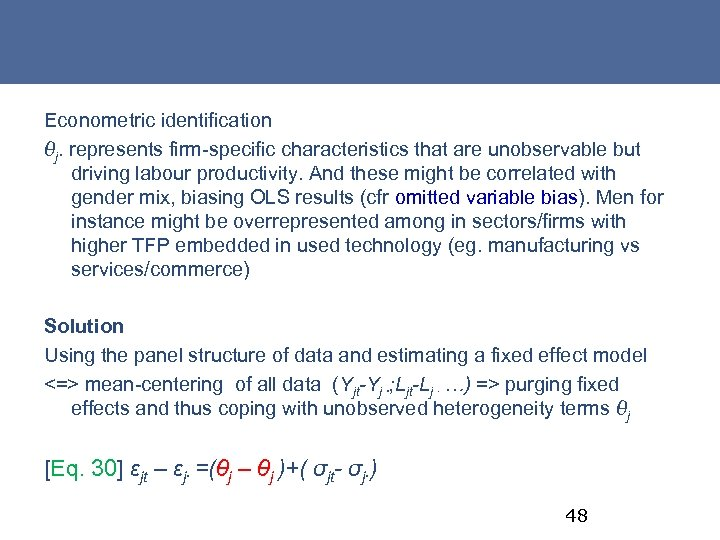Econometric identification θj. represents firm-specific characteristics that are unobservable but driving labour productivity. And these might be correlated with gender mix, biasing OLS results (cfr omitted variable bias). Men for instance might be overrepresented among in sectors/firms with higher TFP embedded in used technology (eg. manufacturing vs services/commerce) Solution Using the panel structure of data and estimating a fixed effect model <=> mean-centering of all data (Yjt-Yj ∙; Ljt-Lj ∙ …) => purging fixed effects and thus coping with unobserved heterogeneity terms θj [Eq. 30] εjt – εj∙ =(θj – θj )+( σjt- σj∙) 48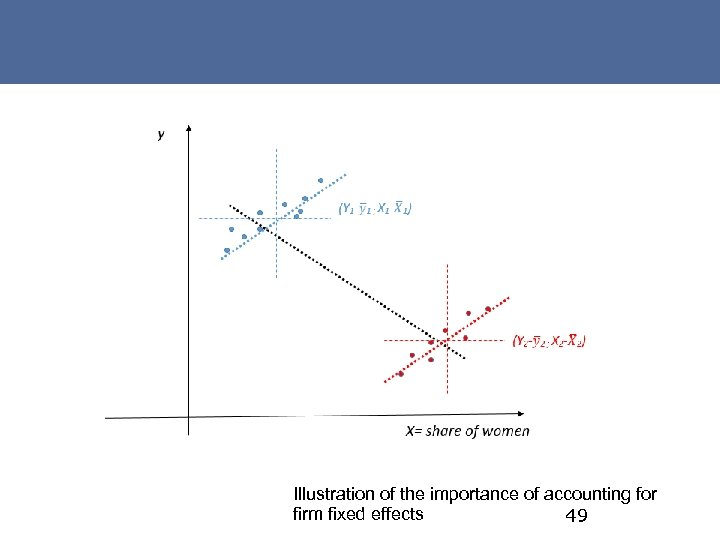Illustration of the importance of accounting for firm fixed effects 49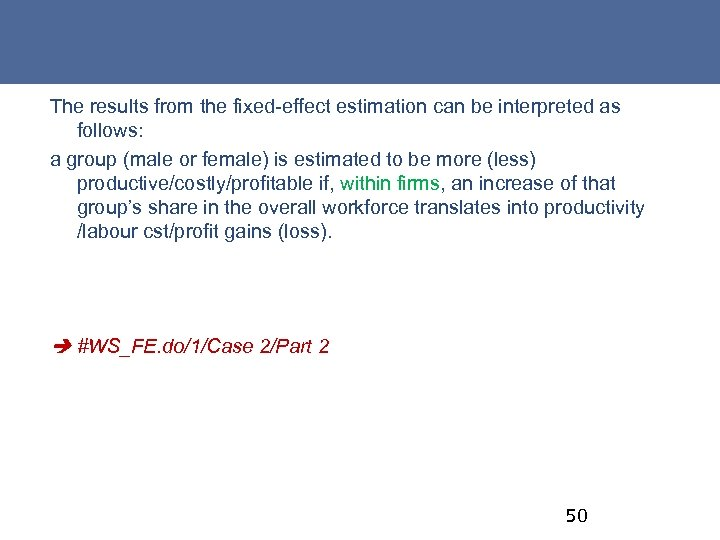The results from the fixed-effect estimation can be interpreted as follows: a group (male or female) is estimated to be more (less) productive/costly/profitable if, within firms, an increase of that group’s share in the overall workforce translates into productivity /labour cst/profit gains (loss). #WS_FE. do/1/Case 2/Part 2 50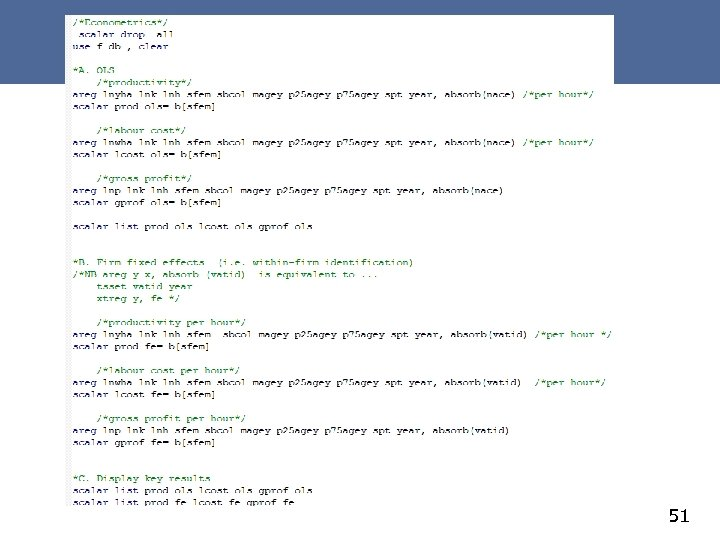51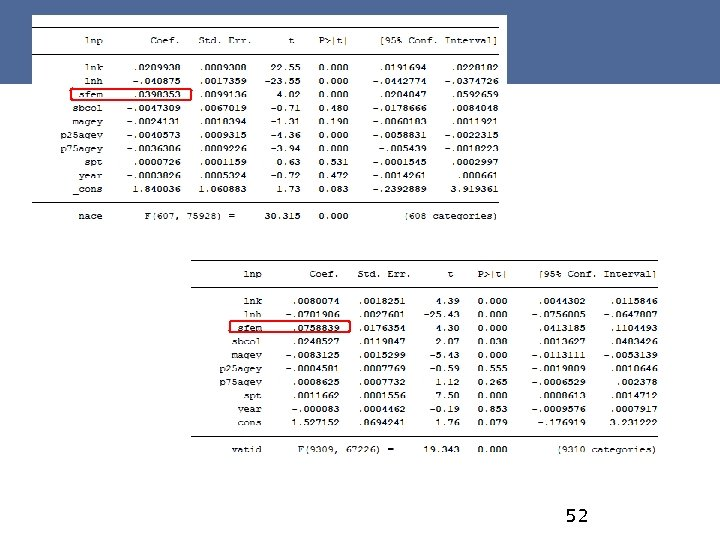52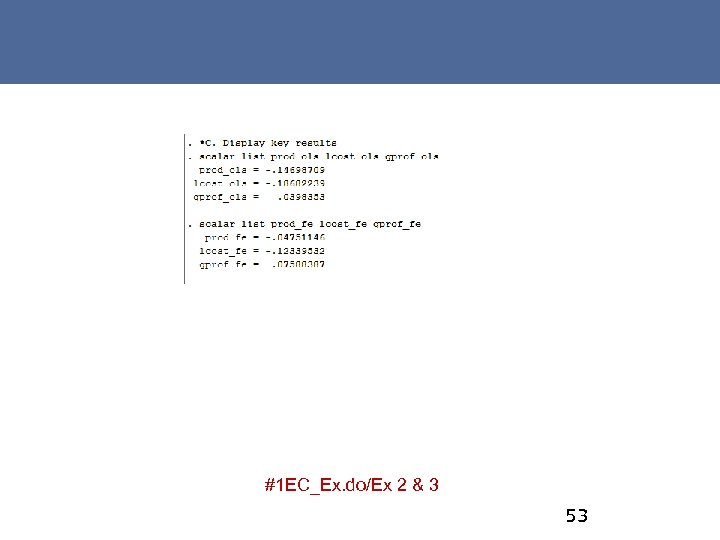#1 EC_Ex. do/Ex 2 & 3 53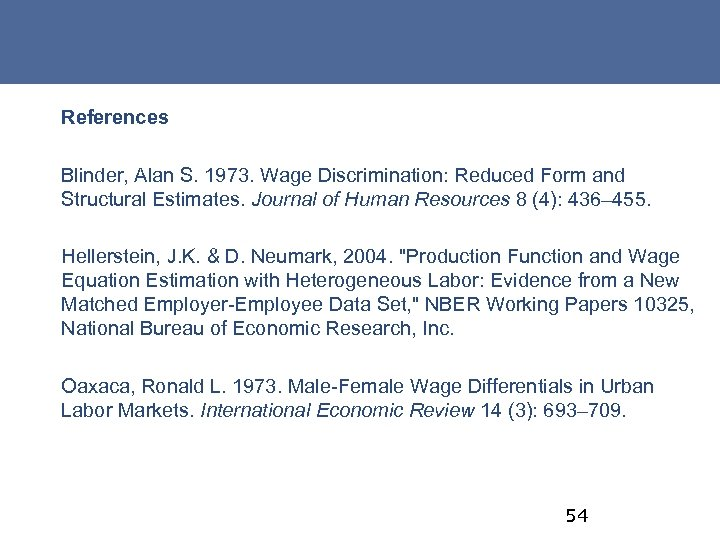References Blinder, Alan S. 1973. Wage Discrimination: Reduced Form and Structural Estimates. Journal of Human Resources 8 (4): 436– 455. Hellerstein, J. K. & D. Neumark, 2004. "Production Function and Wage Equation Estimation with Heterogeneous Labor: Evidence from a New Matched Employer-Employee Data Set, " NBER Working Papers 10325, National Bureau of Economic Research, Inc. Oaxaca, Ronald L. 1973. Male-Female Wage Differentials in Urban Labor Markets. International Economic Review 14 (3): 693– 709. 54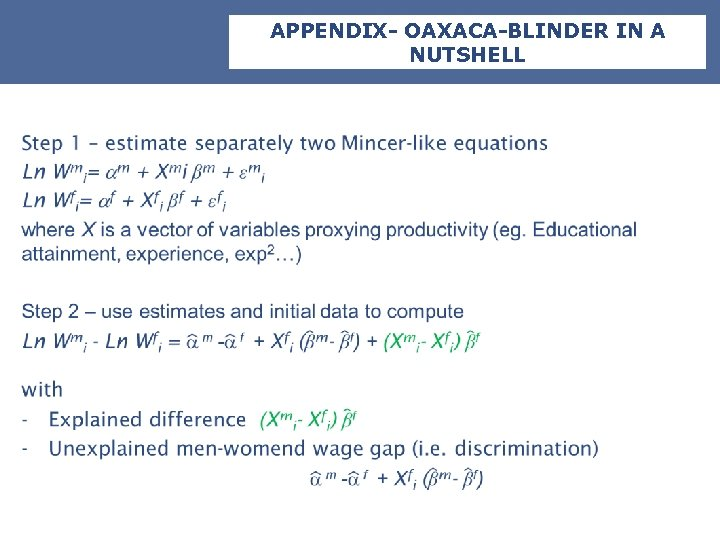APPENDIX- OAXACA-BLINDER IN A NUTSHELL